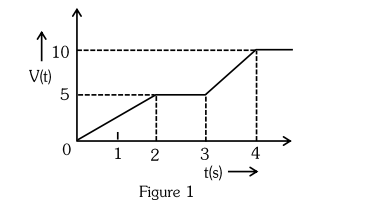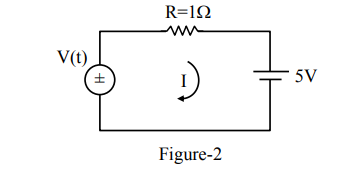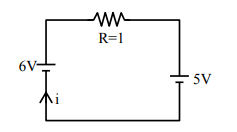# For the circuit shown, the value of current at time t = 3.2 s will be ______ A.`
Question:

For the circuit shown, the value of current at time t = 3.2 s will be ______ A.[Voltage distribution V(t) is shown by Fig. (1) and the circuit is shown in Fig. (2)]

Solution:

From graph voltage at t = 3.2 sec is 6 volt.$i=\frac{6-5}{1}$

$\mathrm{i}=1 \mathrm{~A}$